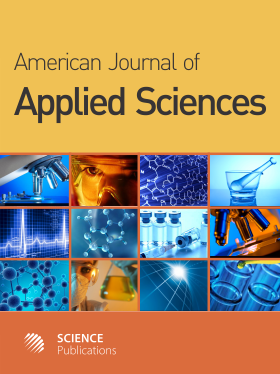Frequency: Continuous
ISSN: 1546-9239 (Print)
ISSN: 1554-3641 (Online)
Research Article Open Access

# Parallel Iterative Algorithms with Accelerate Convergence for Solving Implicit Difference Equations

Qing-Fu Liu1, Wei-Jun Zhong1 and Mancho Manev1
• 1 ,
American Journal of Applied Sciences
Volume 1 No. 1, 2004, 54-61

DOI:

Submitted On: 31 January 2005 Published On: 31 March 2004

How to Cite: Liu, Q., Zhong, W. & Manev, M. (2004). Parallel Iterative Algorithms with Accelerate Convergence for Solving Implicit Difference Equations. American Journal of Applied Sciences, 1(1), 54-61. https://doi.org/10.3844/ajassp.2004.54.61

## Abstract

This study focuses on the solution of implicit difference equations, which are very difficult to compute in parallel for the diffusion equation. For improving the convergence rates and the properties of gradual-approach convergence of Segment-Classic-Implicit-Iterative (SCII) and Segment-Crank-Nicolson-Iterative (SCNI) algorithms realizing efficient iterative computation in parallel by segmenting grid domains, SCII and SCNI algorithms with accelerated convergence are studied and improved through inserting classic implicit schemes and Crank-Nicolson schemes into them respectively. The SCII and SCNI algorithms with accelerated convergence, which can be decomposed into smaller strictly tri-diagonally dominant subsystems, are solved by using a double - sweep algorithm. In the present study, general structures of SCII and SCNI algorithms with accelerated convergence are constructed with matrix forms. The convergent rates are estimated and properties of gradual-approach convergence about diffusion equation are described by a splitting coefficient matrix in detail. These algorithms improve the convergence rates in iteration while making the properties of gradual-approach convergence reach two ranks. The efficiency of computation is greatly enhanced. In addition, the algorithms are extended to the case of two-dimensional problem by studying Peaceman-Rachford scheme into which classic implicit schemes are inserted alternately. Numerical computations employing SCII and SCNI algorithms with accelerated convergence are made to SGL/Challenge L with 8 CPUs as examples. Theoretical analyses and numerical exemplifications show that the parallel iterative algorithms with accelerated convergence for solving one-dimensional diffusion equations are more efficient in computation and have much better convergent rates and properties of gradualapproach convergence.

• 1,150 Views